﻿ Square/Triangular Wave Generator Using Single DO-DVCC and Three Grounded Passive Components

### Square/Triangular Wave Generator Using Single DO-DVCC and Three Grounded Passive Components

Hung-Chun ChienOPEN ACCESSPEER-REVIEWED

## Square/Triangular Wave Generator Using Single DO-DVCC and Three Grounded Passive Components

Hung-Chun ChienDepartment of Electronic Engineering, Jinwen University of Science and Technology, New Taipei City, Taiwan

### Abstract

This study proposes a novel square/triangular wave generator based on a dual outputs differential voltage current conveyor (DO-DVCC). The proposed circuit uses one DO-DVCC combined with three grounded passive components. The presented scheme reveals a compact topology and can produce square/triangular waveform simultaneously. To verify their feasibility, commercially available ICs were used for implementing the prototype circuits. Experimental results revealed consistency with theoretical analyses.

### At a glance: Figures

12
Prev Next

• Chien, Hung-Chun. "Square/Triangular Wave Generator Using Single DO-DVCC and Three Grounded Passive Components." American Journal of Electrical and Electronic Engineering 1.2 (2013): 32-36.
• Chien, H. (2013). Square/Triangular Wave Generator Using Single DO-DVCC and Three Grounded Passive Components. American Journal of Electrical and Electronic Engineering, 1(2), 32-36.
• Chien, Hung-Chun. "Square/Triangular Wave Generator Using Single DO-DVCC and Three Grounded Passive Components." American Journal of Electrical and Electronic Engineering 1, no. 2 (2013): 32-36.

 Import into BibTeX Import into EndNote Import into RefMan Import into RefWorks

### 1. Introduction

In early active RC circuit designs, operational amplifiers (OPAs) played a crucial role in implementing numerous signal generation/processing circuits . Recently, active RC circuit designs using current-mode active devices were attractive because of their potential advantages over traditional OPAs, such as wider bandwidth, higher accuracy, and simplicity in implementation . Current conveyor (CC) was the first introduced current-mode active device, which was reported in 1968 . Since then, several novel active devices have been reported [4-10]. Square/triangular wave generators have wide applications in instrument, measurement, communication, and power conversion circuit control systems. For several years, the typical configuration of square/triangular generator was realized by using OPAs combined with external passive components . In addition to OPA-based configuration, previous studies presented several implementations of square/triangular wave generators using different types of active devices [11-16]. For the reported square/triangular wave generators, one current tunable topology was designed using three operational transconductance amplifiers (OTAs) . This circuit was built based on a current tunable Schmitt trigger connected to an OTA-C integrator. Although OTA-based wave generator exhibits the current tunable property, the transconductance gain of an OTA is a function of temperature. Thus, the wave generators implemented by OTA is sensitive with environmental variation. Moreover, a CC-based square/triangular wave generator was first introduced in 2000 ; and a recent study revealed a modified topology to enhance circuit performance and reduce the passive component counts . A square/triangular wave generator using current feedback amplifiers (CFOAs) has also been reported in . In 2007 literature  presented an operational transresistance amplifier (OTRA) based scheme that featured switch-controllable operation. Recent research reports a DVCC-based topology constructed with two DVCCs and four passive components . This is the first reported square/triangular wave generator built by DVCCs. The concept of the DVCC device was first introduced in 1989 . Because the DVCC-based application circuits received considerable attention in recent years, this study proposes a novel circuit configuration to add to this list. Although DVCC-based square/triangular wave generator was discussed in a previous study , this paper presents a compact topology to implement a low cost design. To illustrate the novelty and difference of the proposed circuit, comparisons with other schemes are shown in Table 1. It should be noted that among the various solutions, the proposed circuit features the following benefits: 1) Fewer of active devices and passive components are used. 2) All passive components are grounded connections. 3) Higher operation frequency than OPA- and OTA-based designs. 4) Insensitive to the temperature.

### 2. DO-DVCC Fundamental and Its Realization

The circuit symbol of a DO-DVCC is shown in Figure 1, which includes two high-impedance voltage input terminals (Y1 and Y2), one low-impedance current output terminal (X), and a couple of high-impedance current output terminals (Z+) Its terminal relationships are defined by (1), where the X terminal voltage follows the voltage difference of terminals Y1 and Y2, and a current injected into the terminal X is replicated to the terminal Z+ of the same flow direction. An ideal DO-DVCC exhibits zero input resistance at the terminal X, and infinite resistances at Y1 and Y2 terminals, as well as at the terminal Z+.

#### Table 1. Comparisons among various square/triangular wave generatorsDownload asPowerPoint Slide

Veiw figureView current table in a new window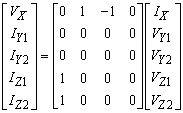(1)

To evaluate the effectiveness of the proposed circuit, a practical implementation of DO-DVCC using commercially available IC (AD844AN) is shown in Figure 2. The AD844AN has the following properties: the voltage on the non-inverting input terminal is transferred to the inverting input terminal, and the current into the inverting input terminal is replicated to the terminal Tz. In this manner, the non-inverting input terminals of the first and second AD844ANs were used to simulate the two high-impedance inputs, Y1 and Y2, of a DO-DVCC, as shown in Figure 2. To produce a terminal voltage Vx proportional to the difference of Y1 and Y2 voltages, a resistor Ra was settled between the inverting input terminals of the first and second AD844ANs, and the Tz node of the first AD844AN was subsequently connected to the non-inverting input terminal of the third AD844AN with a grounded resistor Rb. The fourth and fifth AD844ANs and resistors (Rc, Rd, and Re) perform the function of the dual output currents (Iz1, and Iz2). As shown in Figure 2, the following relationships can be obtained: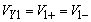(2)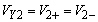(3)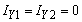(4)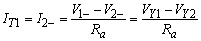(5)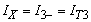(6)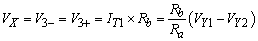(7)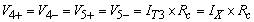(8)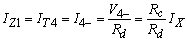(9)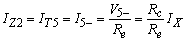(10)

Therefore, if Ra = Rb and Rc = Rd = Re, the terminal behavior of an ideal DO-DVCC can be precisely fulfilled. Because the AD844AN IC is widely used to implement a variety of analog circuits, the realization shown in Figure 2 is capable of providing a viable method to implement a DO-DVCC in practice.

### 3. Circuit Descriptions and Operations

Figure 3 shows the circuit diagram and its output waveforms associated with the proposed square/triangular wave generator. Only one DO-DVCC and three grounded passive components are required. The Y1-Z+ connection in conjunction with the resistor R2 forms a positive-feedback path, and thus, the DO-DVCC saturates with its voltage levels either at the positive saturation level Vo+ or at the negative saturation level Vo at output Vo1 (Vo+ = | Vo−|). In Figure 3(b), Vo+ and Vo− are the two saturation levels of Vo1, and VTH and VTL represent the upper and lower threshold levels, respectively. The circuit operation can be divided into two modes (on-duty cycle T1, and off-duty cycle T2). In the on-duty cycle, Vo1 is at the positive saturation level Vo+ in the beginning. To establish that the voltage level of Vo1 is Vo+ from the beginning, the current IX must have a stronger positive charge than IZ. Let R2 be greater than R1 to achieve this requirement. At this time, the capacitor C is charged, causing Vo2 to increase linearly. The increasing rate of Vo2 and the expressions of currents IX and IZ are determined in (11) to (13). This state continues until Vo2 reaches the upper threshold level VTH. Subsequently, the circuit leaves on–duty cycle operation into the off-duty cycle.

Figure 3. (a) Circuit diagram of the proposed DO-DVCC-based square/triangular wave generator, and (b) its output waveforms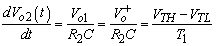(11)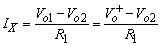(12)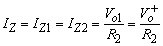(13)

In the off-duty cycle, Vo1 is held at Vo−, and Vo2 starts to decrease linearly. At the end of this discharging state, Vo2 reaches VTL. Subsequently, the operation returns to on-duty cycle mode. The decreasing rate of Vo2 and the currents IX and IZ in this state are expressed in (14) to (16).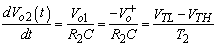(14)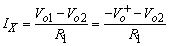(15)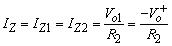(16)

Using (12), (13), (15), and (16), and setting IX = IZ, VTH, and VTL can be derived in (17).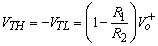(17)

From (11), (14), and (17), the oscillating frequency is provided by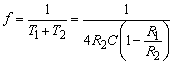(18)

### 4. Non-ideal Effects of the Proposed Circuit

From datasheet , a practical AD844AN IC can be modeled as a positive current conveyor (CC+) cascading a voltage buffer with finite parasitic resistances (Rx, Ry, and Rz) and non-ideal voltage and current tracking gains. A more sophisticated non-ideal model of the applied DO-DVCC (Figure 2) is shown in Figure 4. Parasitic Rx was on the order of several tens of ohms, whereas Ry and Rz were in the range of a few mega ohms. α denotes the non-ideal voltage tracking gain from the non-inverting node to the inverting node, and β represents the non-ideal current tracking gain at Tz with respect to the inverting node of CC+. From the AD844AN datasheet, the standard values of these parameters can be acquired as α = 0.99, β= 0.98, Rx = 50Ω, Ry = 10 MΩ, and Rz = 3 MΩ The resulting expressions of the related currents are included in Figure 4.

Considering the non-ideal DVCC model, for the square/triangular wave generator (Figure 3), the expressions of the increasing/decreasing rate of Vo2 and the currents IX and IZ are modified in (19) to (21).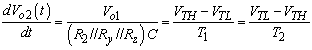(19)(20)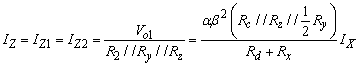(21)

Setting IX = IZ and substituting the saturation levels of Vo1 into (19), (20), and (21), the modified upper and lower threshold levels, VTH and VTL, can be derived as in (22). The oscillating frequency is determined in (23).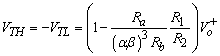(22)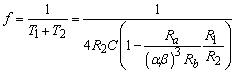(23)

In (22), and (23), the following conditions are applied: R1 >> Rx, R2 << (Ry// Rz), R3 << Rz, Ra >> Rx, Rb << (Ry// Rz), Rc << Ry, Rc << Rz, Rd >> Rx, and Rc= Rd = Re. From (22) and (23), the non-ideal voltage and current tracking gains slightly influenced the threshold levels and oscillating frequency using the presented circuit. However, this slight deviation can be compensated for by tuning the ratio of Ra/Rb. Thus, if (24) is satisfied, the influence of these non-ideal effects would be nearly disregarded.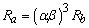(24)

### 5. Design Procedures and Experimental Results

Several experimental tests are presented to demonstrate the theoretical analysis of the proposed circuit. To demonstrate the validity of the theoretical analysis, a prototype circuit was built using AD844AN ICs combined with discrete passive components for experimental testing. All experiments were performed at supply voltages of ±10 V with saturation levels Vo+ = -Vo‾= 9.6 V. Equations (22), (23), and (24) are useful to facilitate the design procedures. First, (24) was applied to fulfill the function of an ideal DO-DVCC. A proper value of Rb was first selected, and parameters α and β were obtained from the AD844AN datasheet. Ra can subsequently be determined. For the proposed square/triangular wave generator (Figure 3), a suitable ratio R1/R2 is chosen, and an oscillating frequency is specified. The capacitor C is arbitrarily determined. Subsequently, R2 and R1 are obtained from (23). Based on the design procedures, substituting the parameters α = 0.99 and β = 0.98 into (24) enables calculation of Ra/Rb = 0.91. To satisfy the conditions Ra >> Rx, and Rb << (Ry // Rz), Ra = 9.1 kΩ and Rb = 10 kΩ were selected. Rc = Rd = Re = 10 kΩ were used to fulfill Rc << Ry, Rc << Rz, Rd >> Rx, and Rc = Rd = Re. For the proposed square/triangular wave generator, the oscillating frequency was specified as f = 10kHz; a resistor ratio R1/R2 = 0.5 was set. Subsequently, C was chosen as 10nF. From (23), R2 = 5kΩ was considerably smaller than Rz //Ry, and R1 = 2.5kΩ was considerably larger than Rx. Figure 5 shows the experimental result of output waveforms for the proposed square/triangular wave generator. It can be concluded that the oscillating frequency for the experimental result was close to the design value f = 10kHz. To investigate the operations for varying oscillating frequencies, the most convenient method was to use a different capacitor, leaving R1 and R2 unchanged. Figure 6 shows the experimental results with f = 100kHz (C = 1 nF), f = 500kHz (C = 200pF), and f = 800kHz (C = 125pF). Because the slew rate of the output voltage for the AD844AN IC and the prototype circuit was implemented on a breadboard, the highest applicable oscillating frequency of the proposed square/triangular wave generator was demonstrated only at approximately several hundred kilohertz, as shown in Figure 6. Obvious distorted output waveforms occurred with an oscillating frequency of 800kHz. The highest operating frequency of the square/triangular wave generators consisting of OPA, and OTA were also verified through the experiment, and were below 100kHz.

Figure 6. Experimental results of output waveforms with (a) f = 100 kHz, (b) f = 500 kHz, (c) f = 800 kHz for the proposed square/triangular wave generator

### 6. Conclusions

This study presents a novel DO-DVCC-based application circuit (DO-DVCC-based square/triangular wave generator). The circuit topology is simple since only one DO-DVCC and a few passive components are utilized. The operation principle of the proposed circuit is described, and the non-ideal effect on the presented circuit is also discussed. The effectiveness of the circuit was verified through experimental tests. Experimental results are consistent with the theoretical analysis. The presented circuits can be widely applied in instrumentation, measurement, communication, and signal processing systems.

### References

  Franco, S, Design with Operation Amplifiers and Analog Integrated Circuits, 3th ed, McGraw-Hall, New York, 2002.In article  Toumazou, C., Lidegy, F. J. and Haigh, D, Analog IC Design: The Current-Mode Approach, Peter Peregrinus Press, U.K, 1990.In article  Smith, K. C. and Sedra, A. S, “The current conveyor: a new circuit building block,” Proceedings of IEEE, 56 (8). 1368-1369. Aug.1968.In article CrossRef  Acar, C. and Ozoguz, S, “A new versatile building block: current differencing buffered amplifier suitable for analog signal processing filter,” Microelectron. J., 30 (2). 157-160. Feb.1999.In article CrossRef  Salama, K. N. and Soliman, A. M, “CMOS operational transresistance amplifier for analog signal processing,” Microelectron. J., 30 (3). 235-245. Mar.1999.In article CrossRef  Alzaher, H. and Ismail, M, “A CMOS fully balanced differential difference amplifier and its applications,” IEEE Trans. Circuits Syst. II, 48 (6). 614-620. Jun.2001.In article CrossRef  Barthelemy, H. and Fabre, A, “A second generation current controlled conveyor with negative intrinsic resistance,” IEEE Trans. Circuits Syst. I, 49 (1). 63-65. Jan.2002.In article CrossRef  Ghallab, Y. H., Badawy, K. K. V. and Maundy, B. J, “A novel current-mode instrumentation amplifier based on operational floating current conveyor,’’ IEEE Transactions on Instrumentation and Measurement, 54 (5). 1941-1949. Oct.2005.In article CrossRef  Maundy, B. J., Sarkar, A. R. and Gift, S. J, “A new design topology for low-voltage CMOS current feedback amplifiers,” IEEE Trans. Circuits Syst. II, 53 (1). 34-38. Jan.2006.In article CrossRef  Siripruchyanun, M. and Jaikla, W, “A current-mode analog multiplier/divider based on CCCDTA,” Int. J. Electron. Commun., 62 (3). 223-227. Mar.2008.In article CrossRef  Almashary, B. and Alhokail, H, “Current-mode triangular wave generator using CCIIs,” Microelectron. J., 31 (4). 239-243. Apr.2000.In article CrossRef  Chung, W. S., Kim, H., Cha, H. W. and Kim, H. J, “Triangular/square-wave generator with independently controllable frequency and amplitude,” IEEE Trans. Instrum. Meas., 54 (1). 105-109. Feb.2005.In article CrossRef  Lo, Y. K. and Chien, H. C, “Switch-controllable OTRA-based square/triangular waveform generator,” IEEE Trans. Circuits Syst. II, 54 (12). 1110-1114. Dec.2007.In article CrossRef  Saque, A. S., Hossain, M. M., Davis, W. A., Russell, H. T. and Carter, R. L, “Design of sinusoidal, triangular, and square wave generator using current feedback amplifier (CFOA),” IEEE Region 5 Conference, 1-5. Apr.2008.In article  Pal, D., Srinivasulu, A., Pal, B. B., Demosthenous, A. and Das, B. N, “Current conveyor-based square/triangular waveform generators with improved linearity,” IEEE Trans. Instrum. Meas., 58 (7). 2174-2180. Jul.2009.In article CrossRef  Minaei, S. and Yuce, E, “A simple schmitt trigger circuit with grounded passive elements and its application to square/triangular wave generator,” Ciruits, Systems, and Signal Processing, 31 (3). 877-888. Jun.2012.In article CrossRef  Pal, K, “Modified current conveyors and their applications,” Microelectron. J., 20 (3). 37-40. Aug.1989.In article CrossRef  Analog Devices AD844 datasheet.In article